# 11-1100/Homework Assignment 2

Jump to: navigation, search

This assignment is due at class time on Thursday, October 20, 2010.

### Solve the following questions

1. (Selick)
1. What it the least integer$n$ for which the symmetric group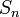$S_n$ contains an element of order 18?
2. What is the maximal order of an element in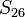$S_{26}$? (That is, of a shuffling of the red cards within a deck of cards?)
2. (Selick) Let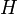$H$ be a subgroup of index 2 in a group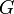$G$. Show that$H$ is normal in$G$.
3. Let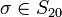$\sigma\in S_{20}$ be a permutation whose cycle decomposition consists of one 5-cycle, two 3-cycles, and one 2-cycle. What is the order of the centralizer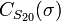$C_{S_{20}}(\sigma)$ of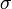$\sigma$?
4. (Selick) Let$G$ be a group of odd order. Show that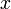$x$ is not conjugate to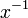$x^{-1}$ unless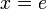$x=e$.
5. (Dummit and Foote) Show that if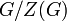$G/Z(G)$ is cyclic then$G$ is Abelian.
6. (Lang) Prove that if the group of automorphisms of a group$G$ is cyclic, then$G$ is Abelian.
7. (Lang)
1. Let$G$ be a group and let$H$ be a subgroup of finite index. Prove that there is a normal subgroup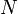$N$ of$G$, contained in$H$, so that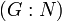$(G:N)$ is also finite. (Hint: Let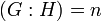$(G:H)=n$ and find a morphism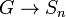$G\to S_n$ whose kernel is contained in$H$.)
2. Let$G$ be a group and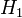$H_1$ and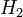$H_2$ be subgroups of$G$. Suppose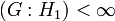$(G:H_1)<\infty$ and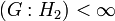$(G:H_2)<\infty$. Show that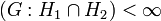$(G:H_1\cap H_2)<\infty$